# The Rayleigh Model

The Rayleigh model is a member of the family of the Weibull distribution. The Weibull distribution has been used for decades in various fields of engineering for reliability analysis, ranging from the fatigue life of deep-groove ball bearings to electron tube failures and the overflow incidence of rivers. It is one of the three known extreme-value distributions (Tobias, 1986). One of its marked characteristics is that the tail of its probability density function approaches zero asymptotically, but never reaches it. Its cumulative distribution function (CDF) and probability density function (PDF) are: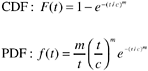where m is the shape parameter, c is the scale parameter, and t is time. When applied to software, the PDF often means the defect density (rate) over time or the defect arrival pattern and the CDF means the cumulative defect arrival pattern.

Figure 7.1 shows several Weibull probability density curves with varying values for the shape parameter m . For reliability applications in an engineering field, the choice of a specific model is not arbitrary. The underlying assumptions must be considered and the model must be supported by empirical data. Of the Weibull family, the two models that have been applied in software reliability are the models with the shape parameter value m = 2 and m = 1.

Figure 7.1. Weibull Probability Density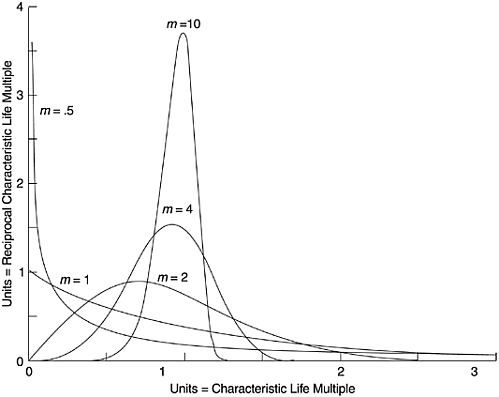The Rayleigh model is a special case of the Weibull distribution when m = 2. Its CDF and PDF are:The Rayleigh PDF first increases to a peak and then decreases at a decelerating rate. The c parameter is a function of t m , the time at which the curve reaches its peak. By taking the derivative of f ( t ) with respect to t , setting it to zero and solving the equation, t m can be obtained.After t m is estimated, the shape of the entire curve can be determined. The area below the curve up to t m is 39.35% of the total area.

The preceding formulas represent a standard distribution; specifically the total area under the PDF curve is 1. In actual applications, a constant K is multiplied to the formulas ( K is the total number of defects or the total cumulative defect rate). If we also substitute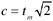in the formulas, we get the following. To specify a model from a set of data points, K and t m are the parameters that need to be estimated.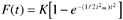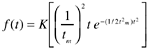It has been empirically well established that software projects follow a lifecycle pattern described by the Rayleigh density curve (Norden, 1963; Putnam, 1978). Early applications of the model in software were mainly for staffing estimation over time for the life cycle of software projects. More recent work demonstrated that the defect removal pattern of software projects also follows the Rayleigh pattern.

In 1982 Trachtenberg (1982) examined the month-by-month error histories of software projects and found that the composite error pattern of those projects resembled a Rayleigh-like curve. In 1984 Gaffney of the IBM Federal Systems Division reported the development of a model based on defect counts at six phases of the development process commonly used in IBM: high-level design inspections, low-level design inspections, code inspections, unit test, integration test, and system test. Gaffney observed that the defect pattern of his data by the six-phase development process followed a Rayleigh curve. Following the system test phase is the phase of field use (customer use). The number of latent defects in the field is the target for estimation.

By developing a Rayleigh model to fit his data, Gaffney was able to project the expected latent defects in the field. Putnam's work includes the application of the Rayleigh model in estimating the number of software defects, in addition to his well-known work on software size and resource estimation (Putnam and Myers, 1992). By validating the model with systems for which defect data are available (including the space shuttle development and radar development projects), Putnam and Myers (1992) found that the total actual defects were within 5% to 10% of the defects predicted from the model. Data fits of a few other systems, for which the validity of the data is doubtful, however, were not so good. As in Trachtenberg's study, the time unit for the Rayleigh model in Putnam and Myers's application is expressed in terms of months from the project start.

Figure 7.2 shows a Rayleigh curve that models the defect removal pattern of an IBM AS/400 product in relation to a six-step development process, which is very similar to that used by Gaffney. Given the defect removal pattern up through system test (ST), the purpose is to estimate the defect rate when the product is shipped: the post general-availability phase (GA) in the figure. In this example the X -axis is the development phase, which can be regarded as one form of logical equivalent of time. The phases other than ST and GA in the figure are: high-level design review (I0), low-level design review (I1), code inspection (I2), unit test (UT), and component test (CT).

Figure 7.2. Rayleigh Model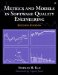Metrics and Models in Software Quality Engineering (2nd Edition)
ISBN: 0201729156
EAN: 2147483647
Year: 2001
Pages: 176